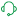# QlikView App Dev

Discussion Board for collaboration related to QlikView App Development.

Announcements
On May 18th at 10AM EDT we will answer your QlikView questions live. REGISTER
cancel
Showing results for
Did you mean:Creator II

## Expression Logic

Hi Team,

I am using 3 List box for the same.

Option1:
Option1
Million (Means will display the value in M)
Full Value (Means Full Value in Integer. like --> 20000000000.25614)
];

Option2:
Option2
'1 M \$'
'2 M \$'
'3 M \$'
];

Option3:
Option3
Yes
No
];

If I select Option1 = 'Million ' and Option2 = '1M\$' and Option3 = 'No'Then I want the sum of Non_PTI, Where Value will be in Million which is Greater Then 1M

If I select Option1 = 'Million ' and Option2 = '2M\$' and Option3 = 'No'Then I want the sum of Non_PTI, Where Value will be in Million which is Greater Then 2M

If I select Option1 = 'Million ' and Option2 = '3M\$' and Option3 = 'No' Then I want the sum of Non_PTI, Where Value will be in Million which is Greater Then 3M

For -->  sum({<account={'EXPENSE'}>}non_ pti)

Else  sum({<account={'EXPENSE'}>}non_ pti_cc) --> This would be also in Million and Greater Than 1M or 2M or 3M As is it previous If selection. Only here Sum value is different -non_ pti_cc

Can You make an expression with these 3 condition  for me.

AK